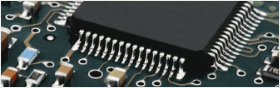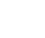• Home
• Instant Online Quote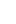• PCB Assembly
• PCB Capabilities
• Feedback
• Resources• About Us#### Dielectric Constant Value For FR-4 Material2019/6/15 15:33:09

The common PCB dielectric constant (refer as DC for the following essay) we use is FR-4, the constant value is 4.2-4.7, which changes with the temperature change. The maximal change may up to 20% within 0-70 degrees centigrade. The DC change will lead to the signal transmission change, The higher the temperature is, the bigger the delay would be. The DC value will also change with signal frequency, the higher the frequency is, the lower the value will be. For any PCB with 100M transmission frequency, we can evaluate the board capacity and delay.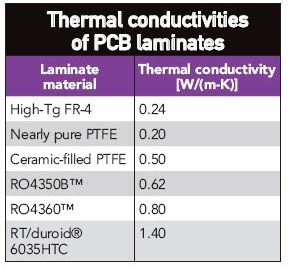The standard inner signal transmission speed is for FR-4 is 180ps/inch(1inch=1000mil=2.54cm),The outer value normally varies from 140 to 170.

The capacity is parallelized with L ,R,C series, there is a resonance point for the capacity. The differences of capacity and technology make the difference of resonance points. Meanwhile, the value is different in different manufacturers.

The resonance points is defined according to the equivalent series inductance. For example, fora100nf capacitor, the point is around 0.5Nh.ESR (equivalent series resistor) value is 0.1ohm,The optimal frequency for filtering is around 24M, the impedance value is 0.1ohm.While for a 1nf capacitor, the relative equivalent series inductance is 0.5nH,with  ESR 0.01ohm. The optimal frequency for filtering is around 200M.To achieve the best filtering function, we will use capacity with a different value.

However, due to the combined function of equivalent series inductance and resistor, there is a resonance point among 24M and 200M, in which there is a maximal impedance control value that bigger than individual capacity one.

Parallel connection of the two capacitors is now equivalent to LC parallel. The sum of the ESR values of the two capacitors is the string resistance of this LC loop. If the LC is connected in parallel, if the series resistance is 0, then there will be an infinite impedance at the resonance point, and there is the worst filtering at this point.

Increasing the ESR will tend to make the overall impedance to a close value. In the frequency band below 24M and in the frequency band above 200M, the impedance will be added, while in the 24M and 200M bands, the impedance will decrease. So just think about the frequency band of the board switching noise. Some foreign planning boards have a few ohms of resistance on a small capacitor (680pF) when the capacitors are connected in parallel, which is probably due to this kind of thinking. (From the above parameters, the on of the 1nF capacitor is 10 times the Q value of the 100nF capacitor. Since there is no detailed equivalent string sense and ESR value from the manufacturer at hand, the above parameters are estimated based on the data seen in the past.

But the error is probably not too big. In the past, the data seen in many places are 1NF and 100nF ceramic capacitors with resonant frequencies of 100M and 10M, respectively. Think about the L of the chip capacitor is much smaller, but did not find a firm value, press it for convenience. 0.5nH accounting. If we have a detailed and reliable value, we also expect to be able to send it up) The dielectric constant (Dk, ε, Er) determines the speed at which the electrical signal is transmitted in the medium. The speed of the electrical signal is inversely proportional to the square root of the dielectric constant.

The lower the dielectric constant, the faster the signal transmission speed. Let's make an image. Just think that you are running on the beach. The water is drowning in your ankle. The viscosity of water is the dielectric constant. The more sticky the water, the higher the dielectric constant and the slower you run.

The dielectric constant is not very simple to measure or define. It is not only related to the characteristics of the medium. but also related to the test method, test frequency, pre-test and data status of the test. The dielectric constant also changes with temperature. Some special data are considered in the development of temperature. Humidity is also an important factor affecting the dielectric constant. Since the dielectric constant of water is 70, there is very little water. Will cause significant changes.

The following are some typical data DC(at 1Mhz): Vacuum 1.0 Pure PTFE 2.1 GY PTFE 2.2-2.3 GX-PTFE 2.55 Cyanate/Glass 3.2 Cyanate/Quartz 2.8-3.4 Polyimide-Quartz 3.5 -3.8 Polyimide-glass 4.0-4.6 Epoxy resin-glass (FR4) 4.4-5.2 Non-woven aromatic amine (aramid) 3.8-4.1 Aromatic amine (woven fabric) 3.8-4.1 Ceramic filled polytetrafluoroethylene 6.0-10.2 Foamclad (Arlon patent) 1.15-1.3 water 70.0.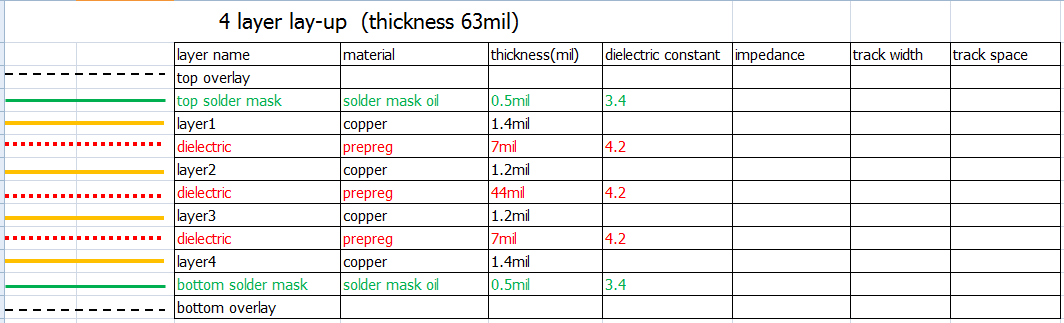It can be seen that for high-speed and high-frequency applications, the most ideal material is an air medium wrapped in copper foil with a thickness tolerance of +/-0.00001". As a data development, we are all in this direction, such as the Arlon patent. The Foamclad developed is very suitable for the use of base station antennas.

But not all planning is the smaller the dielectric constant, the better it is. It is usually based on some practical planning. Some lines with small volume requirements often require high dielectric constant data, such as Arlon's AR1000 for miniaturization. Line planning. Some plans, such as power amplifiers, commonly used dielectric constants of 2.55 (such as Arlon Diclad527, AD255, etc.), perhaps dielectric constant of 3.5 (such as AD350, 25N / FR, etc.) also use 4.5 dielectric constant, such as AD450 From the FR-4 plan to the high-frequency application, and the expectation of the lineage was once planned.

In addition to directly affecting the transmission speed of the signal, the dielectric constant also largely determines the characteristic impedance. In some cases, the characteristic impedance matching is especially important in microwave communication. If the impedance is not matched, the impedance mismatch is also Called VSWR (Standby to Wave Ratio). MAX2242: Printed circuit board data should be FR4 or G-10.

This type of information is an excellent choice for low-cost wireless applications where the operating frequency is below 3 GHz. The MAX2242 evaluation board uses a 4-layer FR4 with a dielectric constant of 4.5 and an insulating layer thickness of 6 mil and 1 oz copper. When planning a low-impedance circuit with an output impedance of approximately (8 j5) at 2.45 GHz like the MAX2242, a 0.5nH inductor can generate an inductive reactance of 8. The inductive reactance of 8 is equivalent to a dielectric constant of 4.5 and a thickness of 6 mils. The impedance of the 60 mil x 10 mil microstrip line on the FR4 printed circuit board.

Here is the formula for resonant frequency: f=1/(2*3.14159*SQRT(L*C), this is the common formula used by the engineer to calculate before the PCB fabrication.##### You might likeAnders Appelgren

7

• Following

0

• Followers

0

PCB PrototypePCB Instant Quote

x mm

Quantity

Quote Now

PCB Assembly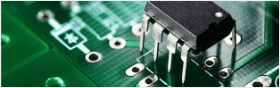SMT-StencilComponents Sourcing